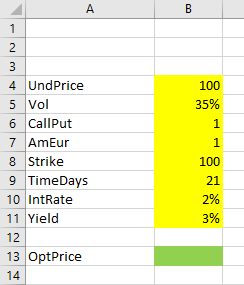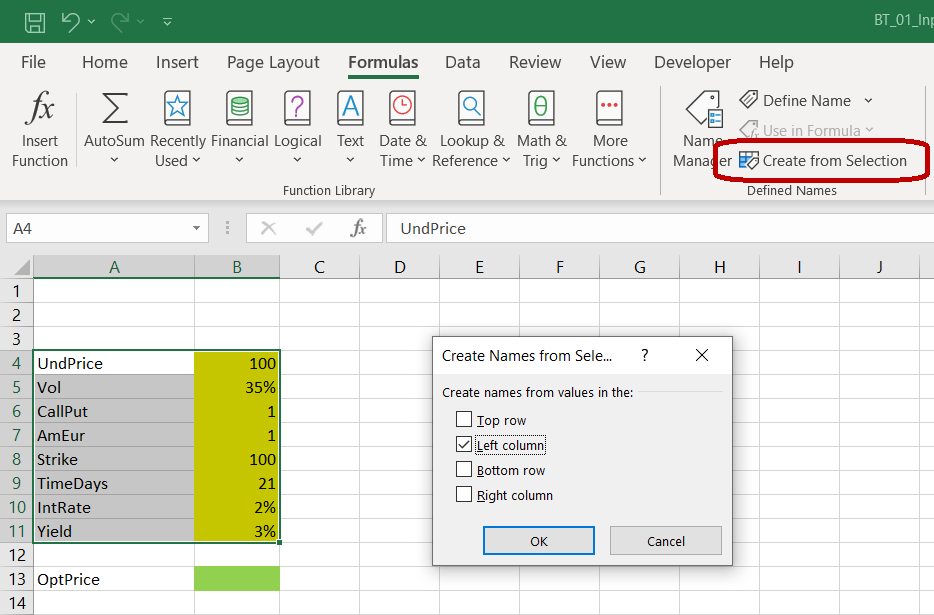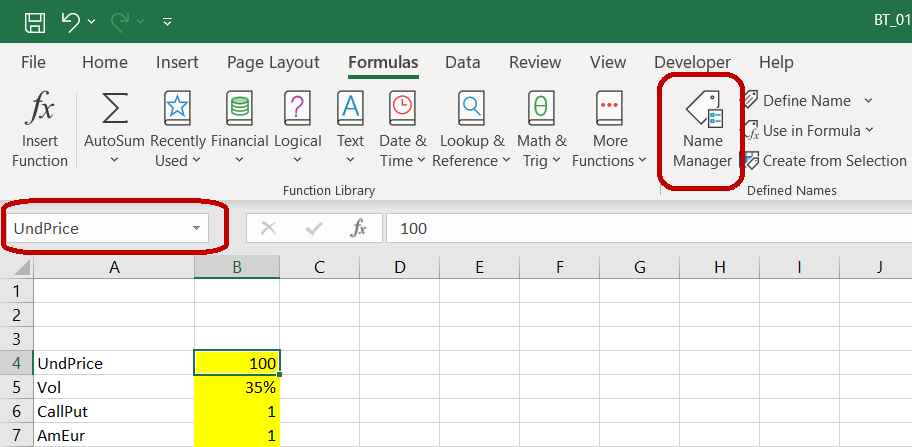# Binomial Option Pricing Excel Tutorial

In this tutorial we will create an option pricing spreadsheet, implementing three popular binomial models: Cox-Ross-Rubinstein, Jarrow-Rudd and Leisen-Reimer.

The spreadsheet will calculate prices of American and European options on stocks, indexes and currencies.

The tutorial has six parts:

2. How binomial trees work (the logic)
3. Creating binomial trees in Excel
4. Cox-Ross-Rubinstein model Excel formulas
5. Jarrow-Rudd model Excel formulas
6. Leisen-Reimer model Excel formulas

If you are already familiar with binomial models and how binomial trees work, you can safely skip part 2.

If not, you will be able to complete the tutorial even if you know nothing about binomial models at the moment. In fact, this hands-on approach is the best way to learn them.

If you are only interested in one of the models, you can skip the parts for the other two models (part 4/5/6).

All three models share the same logic and most of the work is exactly the same (preparing input cells, building binomial trees – covered in parts 1-3). The only part where the models differ is the exact formulas for binomial tree up and down move sizes and probabilities (parts 4/5/6 for individual models).

## Required Excel Skills

You don't need advanced Excel skills to complete this tutorial. You should be comfortable with basic concepts like writing and copying formulas or absolute and relative references.

The hard part of binomial models is the logic and layout of binomial trees; the mathematics is relatively simple. The Excel functions we will use are mostly basic, like `SQRT`, `EXP`, and a lot of `IF`s.

This tutorial will not use VBA and macros.

## Let's Start: Preparing Input Cells

To calculate option prices with binomial models you need a number of inputs, like underlying price, strike price, time to expiration, volatility or interest rate. If you know the Black-Scholes model, you will find the inputs are the same.

It is best to prepare cells for all the inputs right at the beginning, and have all the input cells in one place. It will make the spreadsheet easier to use.

Let's put our inputs in cells `B4-B11` and their labels in column `A`.The inputs are:

• `UndPrice` = current underlying price
• `Vol` = volatility
• `CallPut` = whether the option is a call (1) or put (2)
• `AmEur` = whether the option is American (1) or European (2)
• `Strike` = the option's strike price
• `TimeDays` = time to expiration as number of days; fractional days will also work
• `IntRate` = the risk-free interest rate (domestic rate for currency options)
• `Yield` = continuous dividend yield (for stocks, indexes) or foreign rate (for currency options)

For detailed explanation and which values to use, see Binomial Option Pricing Model Inputs.

The output which we want to calculate is the option price (`OptPrice`) in cell `B13`.

As in other tutorials and calculators, I use yellow background for input cells and green background for output cells.

Note: If you know how to create combo boxes, you can use them for the `CallPut`, `AmEur` inputs. Just make sure call is 1, put is 2, and American is 1, European is 2.

## Naming the Input Cells

To make our formulas easier to write, understand and debug, it is best to name our input cells. It will allow use to write formulas like:

``=MAX(0,IF(CallPut=1,UndPrice-Strike,Strike-UndPrice))``

``=MAX(0,IF(\$B\$6=1,\$B\$4-\$B\$8,\$B\$8-\$B\$4))``

This is how to make it work:

First, make sure your labels in cells `A4-A11` are exactly like mine (`UndPrice`, `Vol` etc.).

Select all the input cells and their labels (the selection is `A4-B11`).

In Excel main menu, go to "Formulas" / "Defined Names" section and click on "Create from Selection".In the window that pops up, check "Left column". This tells Excel to use the contents in the left column (the labels in column `A`) as names for the cells in the right column (the input values in column `B`). Click OK.

Now when you select cell `B4` for instance, the small window on the left of the formula bar is showing "UndPrice" instead of "B4".In formulas, you can now refer to the cell as `UndPrice` instead of `\$B\$4`.

Two notes:

1) You can also set or change cell names one by one by overwriting the text in the cell name window or by using Name Manager (as highlighted in the screenshot above).

2) The navigation may be different in other Excel versions. If you can't find it in yours, google "how to name cells in excel [your version]".

## Next: Binomial Trees

We have our inputs ready and can start working on the calculations. The central part of any binomial option pricing model is the binomial tree, or more precisely, two trees – underlying price tree and option price tree.

In the next part, we will explain how they work (safe to skip if you already know that).

In the part that follows, we will actually create them in our spreadsheet.

By remaining on this website or using its content, you confirm that you have read and agree with the Terms of Use Agreement.

We are not liable for any damages resulting from using this website. Any information may be inaccurate or incomplete. See full Limitation of Liability.

Content may include affiliate links, which means we may earn commission if you buy on the linked website. See full Affiliate and Referral Disclosure.

We use cookies and similar technology to improve user experience and analyze traffic. See full Cookie Policy.